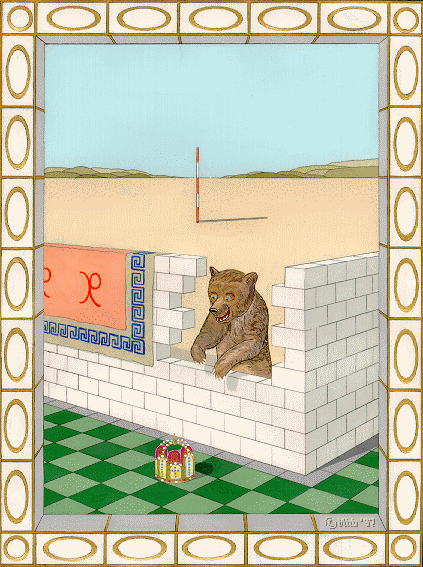The perspective page
(designed for MSIE, requires JavaScript, best view 1024 x 768)
Measurements in the image plane (see: explanation of terms)
Proportions of various distances in the real scene can be determined easily by inversion of the mapping equations.
 xP*: yP*: [in pixels] xP: yP: zP+d: [in metres] measure distance d(V|P) from clickable reference point V from the eye point (V = A) xV: yV: zV+d: [in metres] d(V|P) = m
How the display works:
Each cursor position corresponds to a particular image point P* derived from a real point P.
Line 2 shows the coordinates of P* in pixels (origin of coordinates is the principal point H).
Line 3 shows the coordinates of P in metres (origin of coordinates is the eye point A).
Line 5 Radio switch up: if you click on a point in the image, the corresponding real point V becomes the reference point for measuring a length.
Line 6 Radio switch down: the observer's eye A is the reference point.
Line 7 shows the real coordinates of the reference point in metres.
Line 8 shows the distance in metres from the reference point to the moving real point.
Hint: enable numerical arrow keys to move the cursor precisely.
If coordinates are displayed incorrectly, click the principal point on the horizon 12 pixels away from the right edge of the levelling staff.

English translation by Hugh Casement;   main pageperspective page with auxiliary windowmore paintings with animals